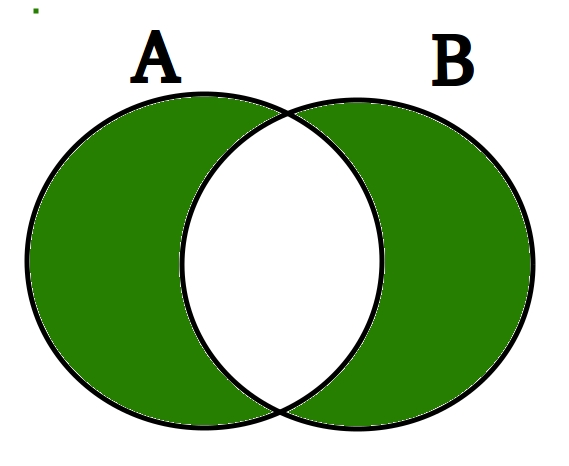Related Articles

# Python set symmetric_difference_update()

• Last Updated : 02 Sep, 2020

The symmetric difference of two sets is the set of elements which are in either of the sets but not in both of them.Symmetric Difference is marked in Green
symmetric_difference() method returns a new set which contains symmetric difference of two sets. The symmetric_difference_update() method updates the set calling symmetric_difference_update() with the symmetric difference of sets.

Syntax:
A.symmetric_difference_update(B)
Parameters:
The symmetric_difference takes a single “iterable” as an argument. Iterable should contain hashable object.
Returns:
This method returns None (which indicates absence of a return value). It only updates the set calling symmetric_difference_update() with the symmetric difference of sets.

CODE 1

## Python

 `# Python code to demonstrate working of``# symmetric_difference_update()` `A ``=` `{``'p'``, ``'a'``, ``'w'``, ``'a'``, ``'n'``}``B ``=` `{``'r'``, ``'a'``, ``'o'``, ``'n'``, ``'e'``}` `# result is always none.``result ``=` `A.symmetric_difference_update(B)` `print``(``'A = '``, A)``print``(``'B = '``, B)``print``(``'result = '``, result)`

Output:

```('A = ', set(['e', 'o', 'p', 'r', 'w']))
('B = ', set(['a', 'r', 'e', 'o', 'n']))
('result = ', None)

```

CODE 2

## Python

 `# Python code to demonstrate working of``# symmetric_difference_update()` `A ``=` `{``'s'``, ``'u'``, ``'n'``, ``'n'``, ``'y'``}``B ``=` `{``'b'``, ``'u'``, ``'n'``, ``'n'``, ``'y'``}` `# result is always none.``result ``=` `A.symmetric_difference_update(B)` `print``(``'A = '``, A)``print``(``'B = '``, B)``print``(``'result = '``, result)`

Output:

```('A = ', set(['s', 'b']))
('B = ', set(['y', 'b', 'u', 'n']))
('result = ', None)

```

CODE 3:

## Python

 `# Python code to demonstrate working of``# symmetric_difference_update()` `A ``=` `{``1``, ``2``, ``3``, ``4``, ``5``, ``6``}``B ``=` `[``4``, ``5``, ``7``, ``8``]` `# passing argument as list` `A.symmetric_difference_update(B)``print``(``"A ="``, A)` `A ``=` `{``2``, ``4``, ``6``, ``8``}``B ``=` `(i ``for` `i ``in` `range``(``2``, ``6``))` `# passing argument as generator object` `A.symmetric_difference_update(B)``print``(``"A="``, A)`
Output
```('A =', set([1, 2, 3, 6, 7, 8]))
('A=', set([3, 5, 6, 8]))

```

CODE 4:

## Python

 `# Python code to demonstrate working of``# symmetric_difference_update()` `A ``=` `{``1``, ``2``, ``3``, ``4``, ``5``}``B ``=` `[[``1``, ``2``, ``3``], ``4``, ``5``]` `# error as b contain one element as list(unhashable object)` `A.symmetric_difference_update(B)``print``(``"A ="``, A)`

Output

```Traceback (most recent call last):
File "/home/1b4e24cadc3fabcd5f90141964a60e9b.py", line 9, in <module>
A.symmetric_difference_update(B)
TypeError: unhashable type: 'list'

```

Attention geek! Strengthen your foundations with the Python Programming Foundation Course and learn the basics.

To begin with, your interview preparations Enhance your Data Structures concepts with the Python DS Course. And to begin with your Machine Learning Journey, join the Machine Learning – Basic Level Course

My Personal Notes arrow_drop_up